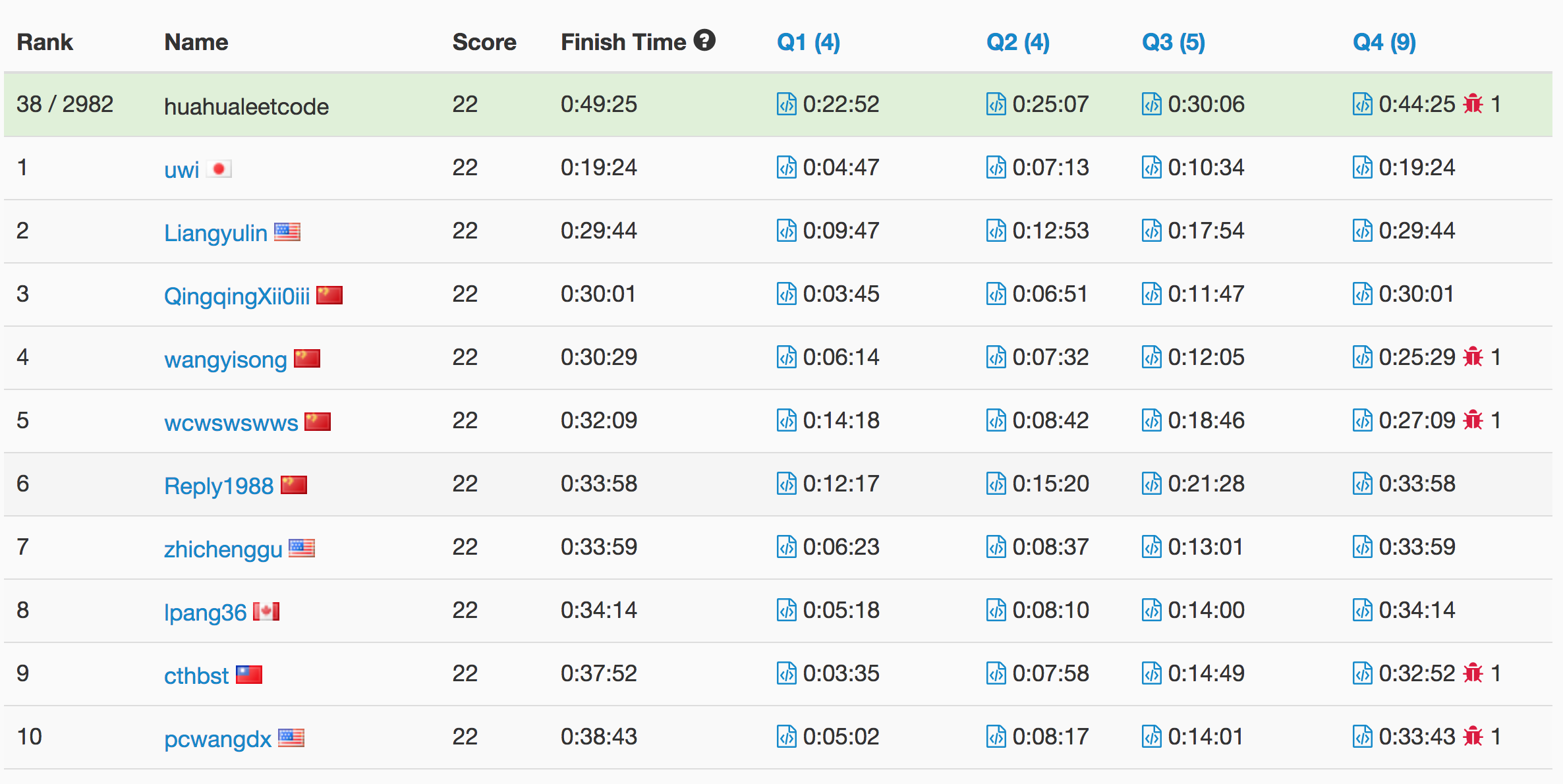# Problem

A website domain like “discuss.leetcode.com” consists of various subdomains. At the top level, we have “com”, at the next level, we have “leetcode.com”, and at the lowest level, “discuss.leetcode.com”. When we visit a domain like “discuss.leetcode.com”, we will also visit the parent domains “leetcode.com” and “com” implicitly.

Now, call a “count-paired domain” to be a count (representing the number of visits this domain received), followed by a space, followed by the address. An example of a count-paired domain might be “9001 discuss.leetcode.com”.

We are given a list cpdomains of count-paired domains. We would like a list of count-paired domains, (in the same format as the input, and in any order), that explicitly counts the number of visits to each subdomain.

Example 1:
Input:
["9001 discuss.leetcode.com"]
Output:
["9001 discuss.leetcode.com", "9001 leetcode.com", "9001 com"]
Explanation:
We only have one website domain: "discuss.leetcode.com". As discussed above, the subdomain "leetcode.com" and "com" will also be visited. So they will all be visited 9001 times.


Example 2:
Input:
["900 google.mail.com", "50 yahoo.com", "1 intel.mail.com", "5 wiki.org"]
Output:
["901 mail.com","50 yahoo.com","900 google.mail.com","5 wiki.org","5 org","1 intel.mail.com","951 com"]
Explanation:
We will visit "google.mail.com" 900 times, "yahoo.com" 50 times, "intel.mail.com" once and "wiki.org" 5 times. For the subdomains, we will visit "mail.com" 900 + 1 = 901 times, "com" 900 + 50 + 1 = 951 times, and "org" 5 times.



Notes:

• The length of cpdomains will not exceed 100.
• The length of each domain name will not exceed 100.
• Each address will have either 1 or 2 “.” characters.
• The input count in any count-paired domain will not exceed 10000.

# Solution: HashTable

Time complexity: O(n)

Space complexity: O(n)

C++

# Problem

https://leetcode.com/problems/valid-square/description/

Given the coordinates of four points in 2D space, return whether the four points could construct a square.

The coordinate (x,y) of a point is represented by an integer array with two integers.

Example:

Input: p1 = [0,0], p2 = [1,1], p3 = [1,0], p4 = [0,1]
Output: True


Note:

1. All the input integers are in the range [-10000, 10000].
2. A valid square has four equal sides with positive length and four equal angles (90-degree angles).
3. Input points have no order.

# Solution

Time complexity: O(1)

Space complexity: O(1)

C++

Write a bash script to calculate the frequency of each word in a text file words.txt.

For simplicity sake, you may assume:

• words.txt contains only lowercase characters and space ' ' characters.
• Each word must consist of lowercase characters only.
• Words are separated by one or more whitespace characters.

For example, assume that words.txt has the following content:

the day is sunny the the
the sunny is is


Your script should output the following, sorted by descending frequency:

the 4
is 3
sunny 2
day 1


Note:
Don’t worry about handling ties, it is guaranteed that each word’s frequency count is unique.

# Ranking

Full list here# Problem

We are to write the letters of a given string S, from left to right into lines. Each line has maximum width 100 units, and if writing a letter would cause the width of the line to exceed 100 units, it is written on the next line. We are given an array widths, an array where widths is the width of ‘a’, widths is the width of ‘b’, …, and widths is the width of ‘z’.

Now answer two questions: how many lines have at least one character from S, and what is the width used by the last such line? Return your answer as an integer list of length 2.

Example :
Input:
widths = [10,10,10,10,10,10,10,10,10,10,10,10,10,10,10,10,10,10,10,10,10,10,10,10,10,10]
S = "abcdefghijklmnopqrstuvwxyz"
Output: [3, 60]
Explanation:
All letters have the same length of 10. To write all 26 letters,
we need two full lines and one line with 60 units.

Example :
Input:
widths = [4,10,10,10,10,10,10,10,10,10,10,10,10,10,10,10,10,10,10,10,10,10,10,10,10,10]
S = "bbbcccdddaaa"
Output: [2, 4]
Explanation:
All letters except 'a' have the same length of 10, and
"bbbcccdddaa" will cover 9 * 10 + 2 * 4 = 98 units.
For the last 'a', it is written on the second line because
there is only 2 units left in the first line.
So the answer is 2 lines, plus 4 units in the second line.


Note:

• The length of S will be in the range [1, 1000].
• S will only contain lowercase letters.
• widths is an array of length 26.
• widths[i] will be in the range of [2, 10].

# Solution: Simulation

Time Complexity: O(n)

Space Complexity: O(1)

Mission News Theme by Compete Themes.